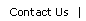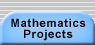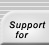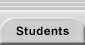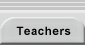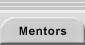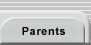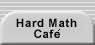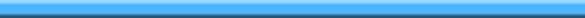Home > Mathematics Projects > Pascal’s Triangle > Warm Up Problems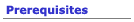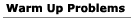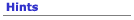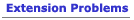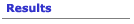## Warm Up Problems for the Patterns in Pascal’s Triangle Problem

1. Describe some of the patterns that you see in Pascal’s triangle. How can you generate new rows of the triangle?
2. The picture below shows the first few steps in the creation of Sierpinski’s carpet: begin with a black square, cut it into 9 congruent squares (as in a tic tac toe game) and remove the central square (or paint it white). For the remaining 8 squares, repeat this process at a smaller scale. For each new step, remove the central square of all remaining black squares (each with dimensions one third as big as in the previous step). After n steps, what fraction of the original square is still black? As we let n get larger and larger, we get ever closer to the fractal known as Sierpinski’s carpet. What percent of Sierpinski’s carpet is black?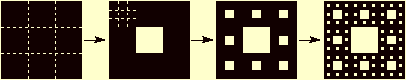3. Do the problems associated with the modular arithmetic resources.
4. Work on the Trains project and its extension problems.
5. Consider the following different definitions: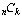= the number of subsets of size k that can be made from an n-element set.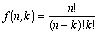Pascal(n, k) = the entries of Pascal’s triangle which follow these two rules:

Pascal(n, k) = Pascal(n – 1, k – 1) + Pascal(n – 1, k)

Pascal(n, 0) = Pascal(n, n) = 1.

a) Write out the first few values ofin a triangle: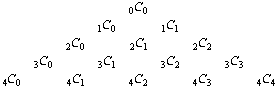b) Write out the first few values of f(n, k) in a triangle: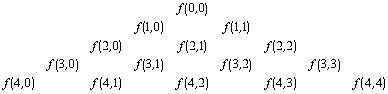c) Write out the first few values of a Pascal(n, k) triangle.

d) Show that for any positive integer n and for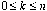, f(n, k) = Pascal(n, k). Hint: you can do this by showing that f(n, k) obeys the same rules that define Pascal(n, k).

e) Show that for any positive integer n and for,= Pascal(n, k).

Because of problems 5d and 5e, we can use the same symbol for, f(n, k), and Pascal(n, k). By convention, most people use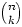.

6. Prove the Binomial Theorem: If n is a non-negative integer,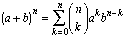This theorem tells us that the coefficients of a binomial expansion are the same numbers that arise from the three functions defined in problem 5 above. Download Binomial Handout for a hint that relates the coefficients to. Alternatively, or additionally (!), you might try to prove the binomial theorem inductively by showing that the binomial coefficients obey the same rules as Pascal(n, k) (hint: how do you computefrom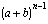?).Translations of mathematical formulas for web display were created by tex4ht. © Copyright 2003 Education Development Center, Inc. (EDC)